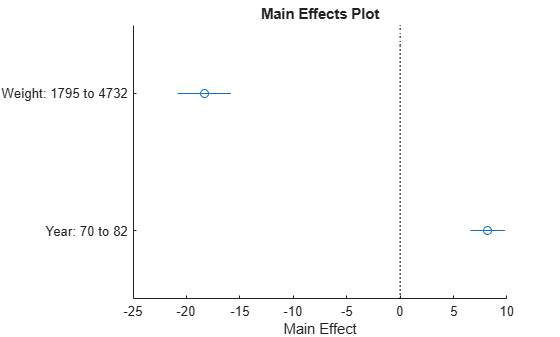# plotEffects

Plot main effects of predictors in linear regression model

## Syntax

``plotEffects(mdl)``
``h = plotEffects(mdl)``

## Description

example

````plotEffects(mdl)` creates an effects plot of the predictors in the linear regression model `mdl`. An effects plot shows the estimated main effect on the response from changing each predictor value, averaging out the effects of the other predictors. A horizontal line through an effect value indicates the 95% confidence interval for the effect value.```
````h = plotEffects(mdl)` returns line objects. Use `h` to modify the properties of a specific line after you create the plot. For a list of properties, see Line Properties.```

## Examples

collapse all

Load the `carsmall` data set and fit a linear regression model of the mileage as a function of model year, weight, and weight squared.

```load carsmall tbl = table(MPG,Weight); tbl.Year = categorical(Model_Year); mdl = fitlm(tbl,'MPG ~ Year + Weight^2');```

Create an effects plot.

`plotEffects(mdl)`The length of each horizontal line in the figure shows a 95% confidence interval for the effect on the response of the change shown for each predictor. For example, the estimated effect of changing `Year` from `70` to `82` is an increase of about 8, and is between 6 and 10 with 95% confidence.

## Input Arguments

collapse all

Linear regression model object, specified as a `LinearModel` object created by using `fitlm` or `stepwiselm`, or a `CompactLinearModel` object created by using `compact`.

## Output Arguments

collapse all

Line objects, returned as a vector. `h(1)` corresponds to the circles that represent the effect estimates, and `h(j+1)` corresponds to the 95% confidence interval for the effect of predictor `j`. Use dot notation to query and set properties of line objects. For details, see Line Properties.

collapse all

### Main Effect

An effect, or main effect, of a predictor represents an effect of one predictor on the response from changing the predictor value while averaging out the effects of the other predictors.

For a predictor variable xs, the effect is defined by

g(xsi) – g(xsj) ,

where g is an Adjusted Response function. The `plotEffects` function chooses the observations i and j as follows. For a categorical variable that is not ordinal, xsi and xsj are the predictor values that produce the maximum and minimum adjusted responses, respectively, so that the effect value is always positive. For a numeric variable or an ordinal categorical variable, the function chooses two predictor values that produce the minimum and maximum adjusted responses where xsi < xsj.

`plotEffects` plots the effect value and the 95% confidence interval of the effect value for each predictor variable.

An adjusted response function describes the relationship between the fitted response and a single predictor, with the other predictors averaged out by averaging the fitted values over the data used in the fit.

A regression model for the predictor variables (x1, x2, …, xp) and the response variable y has the form

yi = f(x1i, x2i, …, xpi) + ri,

where f is a fitted regression function and r is a residual. The subscript i represents the observation number.

The adjusted response function for the first predictor variable x1, for example, is defined as

`$g\left({x}_{1}\right)=\frac{1}{n}\sum _{i=1}^{n}f\left({x}_{1},{x}_{2i},{x}_{3i},...,{x}_{pi}\right),$`

where n is the number of observations. The adjusted response data value is the sum of the adjusted fitted value and the residual for each observation.

`${\stackrel{˜}{y}}_{i}=g\left({x}_{1i}\right)+{r}_{i}.$`

`plotAdjustedResponse` plots the adjusted response function and the adjusted response data values for a selected predictor variable.

## Tips

• The data cursor displays the values of the selected plot point in a data tip (small text box located next to the data point). The data tip includes the x-axis and y-axis values for the selected point. Use the x-axis values to view an estimated effect value and its confidence bounds.

## Version History

Introduced in R2012a